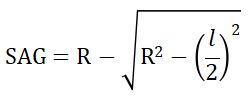# SAG Calculator

This is an online calculator to calculate the SAG value. Just enter the radius of curvature and chord length to get the corresponding SAG value.

• mm
• mm

### Result

• SAG
mm
##### Formula for SAG CalculatorConversion EquationWhere ‘R’ is the Radius of Curvature and ‘𝑙’ is the length of the Chord joining the two vertices of the surface.

SAG - Sagitta, commonly known as Sag is a parameter directly related to the Radius of Curvature of a surface. It is the distance from the exact center of an arc to the center of its base. In optics, Sag is related to either the convex or concave curvature and represents the physical distance between the vertex (highest and lowest point) point along the curve and the center point of a line drawn perpendicular to the curve from one edge of the optic to the other.

Radius of Curvature of a surface is the radius of the circle of which the surface is a part.

Vertex is the point where two or more edges meet that form an angle.